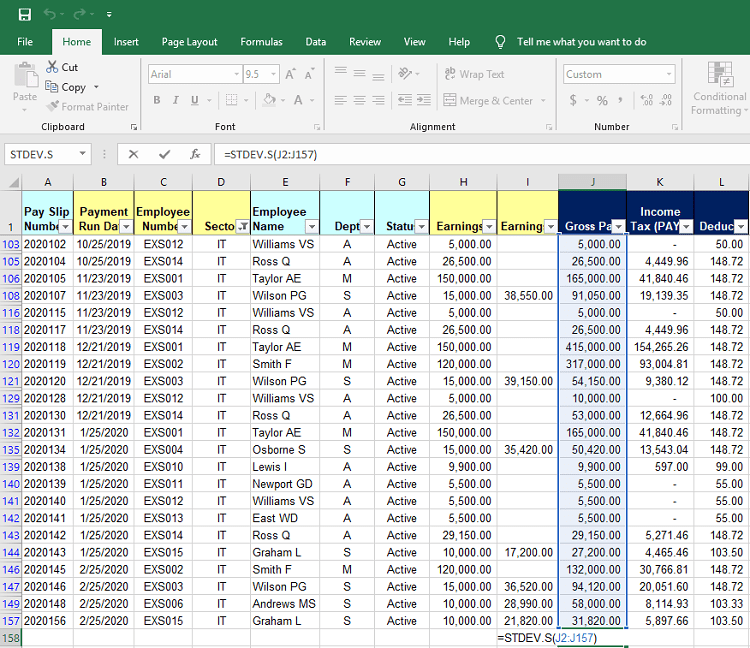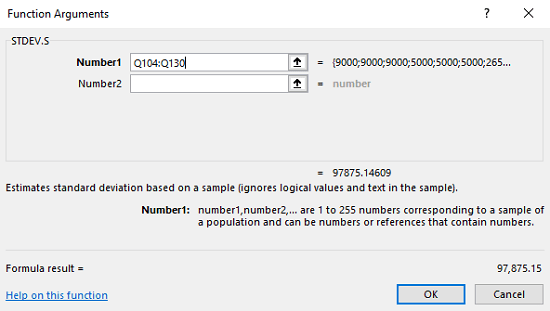0

# How To Calculate Standard Error in ExcelStandard error or standard deviation is an extremely handy tool when you want to gain a deeper understanding of the data that’s in front of you. It tells you how much values in a particular data set deviate from the mean value.

There two main varieties – standard deviation for a sample and standard deviation for a population, and they’re both included in Excel. Let’s see how to go about calculating standard deviation in Excel.

## Standard Deviation for a Sample

Standard deviation for a sample is one of two major standard deviation functions MS Excel lets you calculate for your charts. It represents the standard deviation from the mean for a selected sample of data.

By using this function, you can easily calculate how much a certain subset of data deviates from the mean value. Let’s say that you have a chart with the salaries of all employees in a company and you only want the data on the salaries in the IT sector. You’ll use the standard deviation for a sample or the STDEV.S function.

## Standard Deviation for a Population

Standard deviation for a population is the other major standard deviation function you can calculate through MS Excel. As opposed to the standard deviation for a sample, standard deviation for a population shows the average deviation for all entries in a table. It is marked as STDEV.P in MS Excel.

So, using the same example from the previous section, you would use the STDEV.P function to calculate the deviation for all employees. Excel also lets you calculate other types of standard deviations, though these two are most commonly used. It is worth noting that STDEV.P is the same as the STDEV function.

## How to Calculate Standard Deviation with Excel

Calculating standard deviation in Excel is easy and can be done in three different ways. Let’s take a closer look at each of the methods.

### Method 1

This is the fastest way to calculate the standard deviation value. You can use it to get both the sample and population deviations. However, you need to know the formulas to make this method work, which is why so many people tend to avoid it.

In this case, we’re working with a ten-column chart. Here’s how to do it:

1. Create or open a table in MS Excel.
2. Click on the cell where you’d like the standard deviation value to be displayed.
3. Next, type “=STDEV.P(C2:C11)” or “=STDEV.S(C4:C7)”. The values in the brackets denote the range of cells for which you want to calculate the standard deviation value. In this example, you want to calculate STDEV.P for cells C2 to C11 and STDEV.S for cells C4 to C7.4. Press “Enter”.
5. If you want to round the result to two decimals, click on the “Home” tab.
6. Click the arrow next to “General” to open the dropdown menu.
7. Choose the “Number” option.

### Method 2

The next method is almost as fast as the first one and doesn’t require in-depth Excel knowledge. It is great when you’re in a crunch but don’t want to mess with the formulas. Let’s see how to get the deviations without typing the formulas.

1. Create or open a table in MS Excel.
2. Click on the cell where the deviation result will appear.
4. After that, click the “Insert Function” button. It is located on the left side.
5. Click the arrow next to “Or select category” to open the dropdown menu.
6. Select “Statistical”.7. Browse the list below and select either STDEV.P or STDEV.S
8. Next, in the “Function Arguments” window, enter the range for which you want to calculate the standard deviation into the text box next to “Number1”. Going back to the Method 1 example where we were calculating the standard deviation for cells C2 to C11, you should write C2:C11.When you calculate the standard deviation this way, you won’t need to trim the number, as it will automatically be trimmed to two decimals.

### Method 3

There is also a third method, the one that involves the use of Excel’s Data Analysis toolkit. If you don’t have it, here’s how to install it.

1. Click on “File”.
2. Next, click on “Options”.
3. Click the “Add-Ins” tab on the left side of the window.
4. Click the “Go” button near the bottom of the window.5. Check the “Analysis ToolPak” box.
6. Click “OK”.

With the installation complete, let’s see how to use Data Analysis to calculate Standard Deviation.

1. Create or open a table in MS Excel.
2. Click on the “Data” tab.
3. Select “Data Analysis”.
4. Select “Descriptive Statistics”.
5. In the “Input Range” field, insert the range of cells you wish to include.
6. Choose between the “Columns” and “Rows” radio buttons.
7. Check “Labels in First Row” if there are column headers
8. Pick where you want the result to appear.
9. Check the “Summary Statistics” box.
10. Click the “OK” button.

You will find the standard deviation for population in the output summary.

## Summary

The standard error or standard deviation value can be calculated in a number of ways. Choose the method that suits you best and follow the steps laid out in this article.

Disclaimer: Some pages on this site may include an affiliate link. This does not effect our editorial in any way.